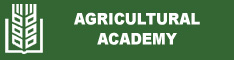Dependencies between quantitative traits, determining the seed productivity in white lupine accession
Valentin Kosev and Natalia Georgieva
Abstract: The experiment was conducted during the period 2016-2018 at the Institute of Forage Crops (Pleven, Bulgaria) to establish the phenotypic and genotypic correlations between the main quantitative traits in 15 accessions of white lupine. Correlation and regression analyzes were applied. The results showed that the values of the positive genotypic correlations in the studied white lupine accessions were higher than the ones of phenotypic correlations for all traits, whereas in negative coefficients the trend was reversed. The genotypic correlation of seeds number with seed weight (r=0.974; r=0.901; r=0.832) and pods number per plant (r = 0.980; r = 0.881) was strong and positive. Positive were the genotypic relationships of plant height with 1st pod height (r = 0.632; r = 0.635; r = 0.895), and pod length with 1000 seeds mass (r = 0.655; r = 0.559; r = 0.382). The values of the correlation coefficients for plant height with 1st pod height, pods with seeds number, 1000 seeds mass with seed weight and pod length, and seeds number with seed weight remained relatively constant during the three years of the study. Positive share for the formation of seed productivity had the traits of seeds number of per plant (R = 0.200; R = 0.253; R = 0.240) and 1000 seeds mass (R = 0.080; R = 0.044; R = 0.018). Seeds number and 1000 seeds mass had direct positive effect on the seeds weight per plant, and seeds and pods number and plant height had the highest total effect.
Keywords: accessions; correlations; dependence; productivity; trait; white lupine
Date published: 2022-07-04